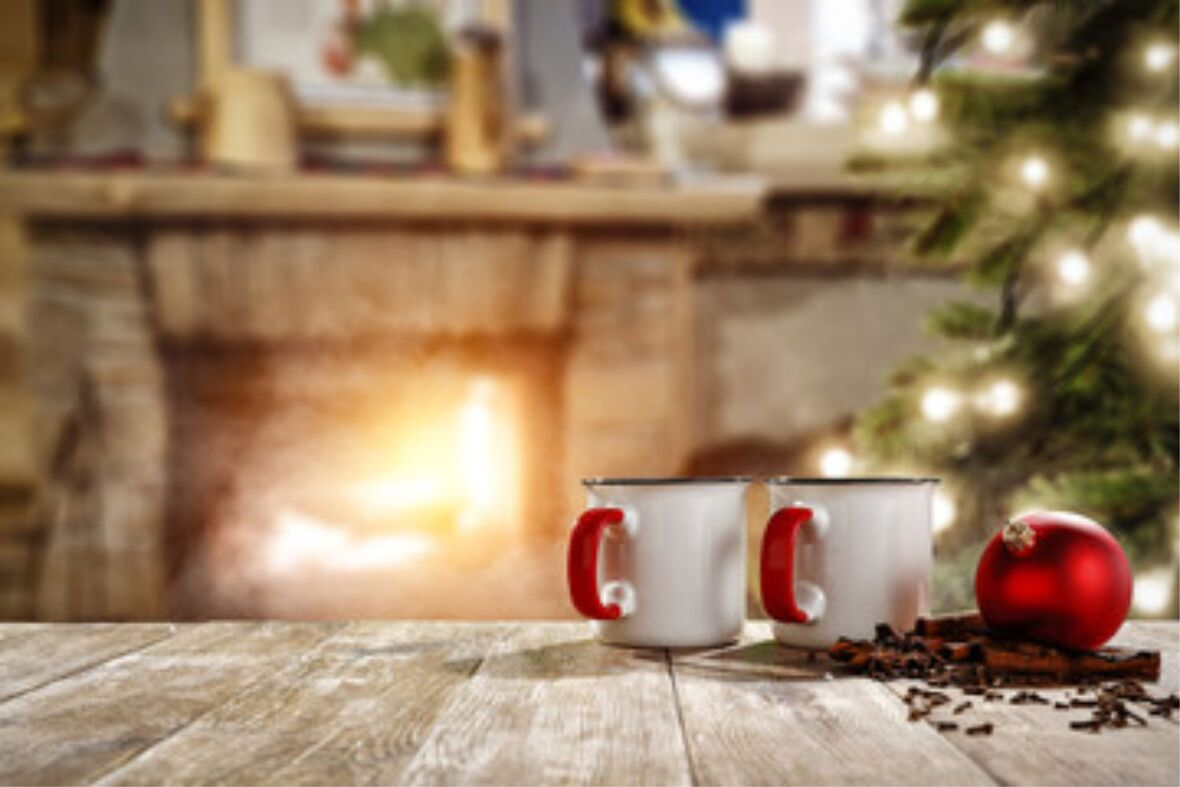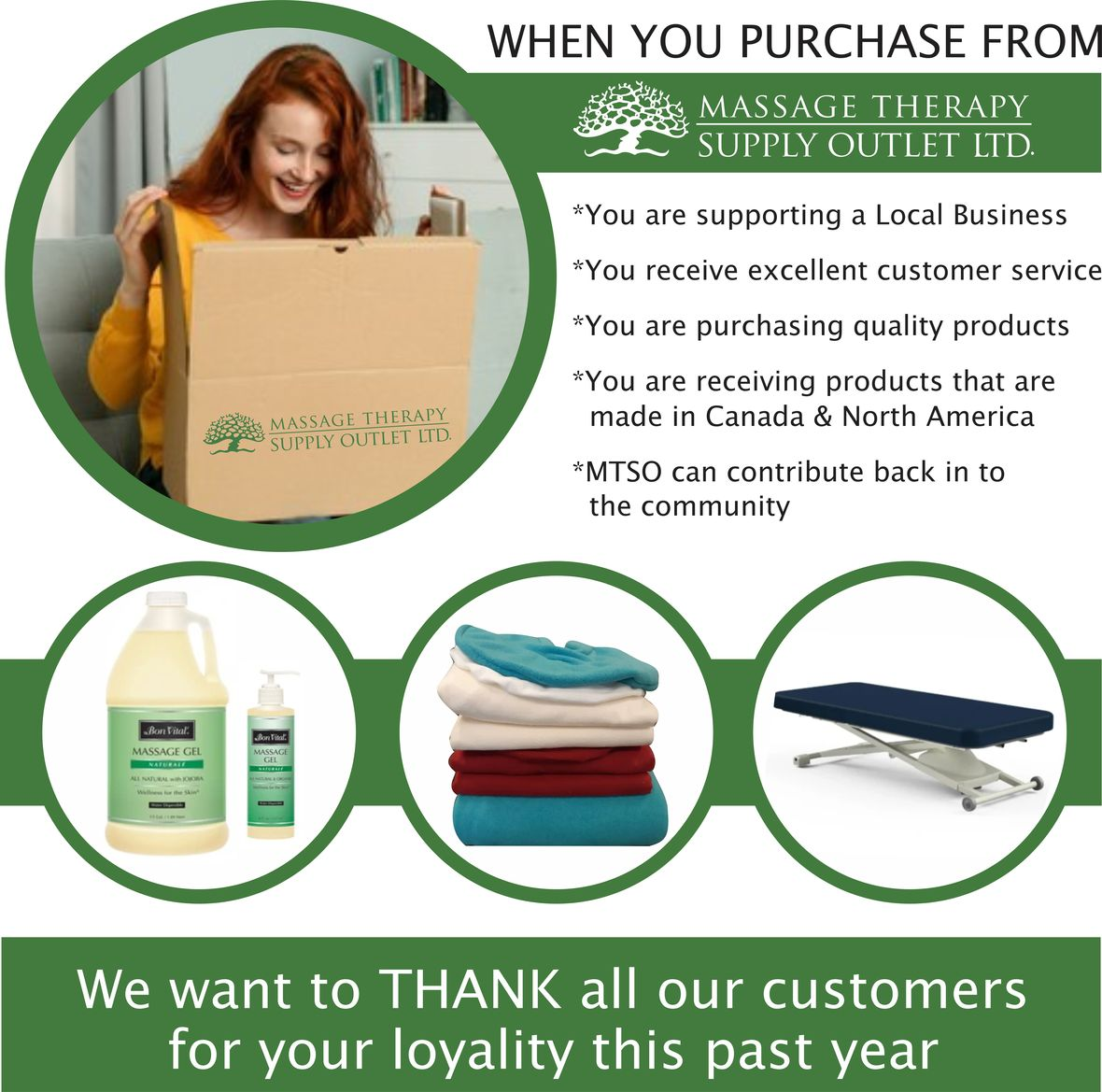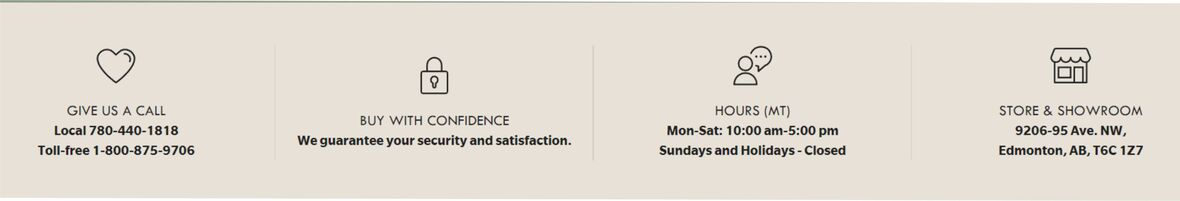## MTSO December NewsNow that the excitements of the Festive Season gather speed & sparkle around us, remember self-care. Maybe seek out your favourite massage therapist for a session or exchange treatments with gift cards for the New Year!

Here are some simple Essential Oil Holiday Blends to freshen the air & add cheer. We also are sharing products that will keep your clients warm and cozy.

As this year draws to a close, please accept our sincere thanks for your loyal ongoing support of our small local business.

From all of us at MTSO Ltd., we wish you a wonderful Holiday Season & a Happy 2023 of renewed prosperity!

 table div table+table+table+table div table{width:100%;padding:0}table div table+table+table+table div table img{width:96.23%;padding:0;float:none}table div table+table+table+table div table td{width:100%;padding:0 1.88% 18px}/* styles */table div table+table+table+table+table+table+table div table{width:100%;padding:0}table div table+table+table+table+table+table+table div table img{width:96.23%;padding:0;float:none}table div table+table+table+table+table+table+table div table td{width:100%;padding:0 1.88% 18px}/* styles */## Holiday Aromatherapy

 table div table+table+table+table+table+table+table+table+table div table{width:100%;padding:0}table div table+table+table+table+table+table+table+table+table div table table{padding:0;float:left!important;width:51.886%!important}table div table+table+table+table+table+table+table+table+table div table table+table td,table div table+table+table+table+table+table+table+table+table div table td{padding-left:0;padding-right:0}table div table+table+table+table+table+table+table+table+table div table table td{padding-left:0;padding-right:20px}table div table+table+table+table+table+table+table+table+table div table table+table{float:left!important;width:48.114%!important}/* styles */

## Our favourite seasonal blends

You'll love the beneficial effects of any of these delightful holiday blends - transform your space with nature's aromatic essences.

Link HERE to our essential oils for all the ingredients.

 table div table+table+table+table+table+table+table+table+table+table+table div table{width:100%;padding:0}table div table+table+table+table+table+table+table+table+table+table+table div table img{width:96.23%;padding:0;float:none}table div table+table+table+table+table+table+table+table+table+table+table div table td{width:100%;padding:0 1.88% 18px}/* styles */\$157.00
Feel the quality. Feel the comfort. Feel the difference. Oakworks Premium Table Warmer

▪ Full Size: 72"x 30" fits most tables
▪ Distributes heat evenly
▪ Programmable temperature
▪ settings from 86° to an incredible 130°
▪ Continuous real time temperature read out
▪ Rapid warming feature
▪ Programmable auto shut-off feature, (up to 99 minutes)
 ▪ Full Size: 72"x 30" fits most tables
 ▪ Distributes heat evenly
 ▪ Programmable temperature
 ▪ settings from 86° to an incredible 130°
 ▪ Continuous real time temperature read out
 ▪ Rapid warming feature
 ▪ Programmable auto shut-off feature, (up to 99 minutes)
 table div table+table+table+table+table+table+table+table+table+table+table+table+table+table div table{width:100%;padding:0}table div table+table+table+table+table+table+table+table+table+table+table+table+table+table div table img{width:96.23%;padding:0;float:none}table div table+table+table+table+table+table+table+table+table+table+table+table+table+table div table td{width:100%;padding:0 1.88% 18px}/* styles */# ELECTRIC TABLE WARMER DELUXE

\$153.00
NOW WITH REMOVEABLE AND WASHABLE SHERPA COVER!!

Massage Table Warmer

Provides gentle and comfortable warmth during massages.

Full size (33"x 73") table warmer quickly and evenly distributes the heat.

▪ Ultra Soft and luxurious fleece pad
▪ 2 table positioning straps on either end
▪ 5 temperature settings
▪ Timer setting 1-10
▪ Auto overheating protection
 ▪ Ultra Soft and luxurious fleece pad
 ▪ 2 table positioning straps on either end
 ▪ 5 temperature settings
 ▪ Timer setting 1-10
 ▪ Auto overheating protection

Thickly padded quilted top gives extra comfort.

 table div table+table+table+table+table+table+table+table+table+table+table+table+table+table+table+table+table div table{width:100%;padding:0}table div table+table+table+table+table+table+table+table+table+table+table+table+table+table+table+table+table div table img{width:96.23%;padding:0;float:none}table div table+table+table+table+table+table+table+table+table+table+table+table+table+table+table+table+table div table td{width:100%;padding:0 1.88% 18px}/* styles */# PROFESSIONAL STONE HEATER

\$180.00
Clients absolutely love a hot stone massage! Hot stones can even be used during pedicures, manicures, or other spa treatments for a special added touch. Our Deluxe Hot Stone Warmers will ensure your stones are heated evenly and safely. This model comes with a free thermometer and wooden slotted spoon.

 table div table+table+table+table+table+table+table+table+table+table+table+table+table+table+table+table+table+table+table+table div table{width:100%;padding:0}table div table+table+table+table+table+table+table+table+table+table+table+table+table+table+table+table+table+table+table+table div table img{width:96.23%;padding:0;float:none}table div table+table+table+table+table+table+table+table+table+table+table+table+table+table+table+table+table+table+table+table div table td{width:100%;padding:0 1.88% 18px}/* styles */table.module-20{width:89.06%;padding:0}table div table+table+table+table+table+table+table+table+table+table+table+table+table+table+table+table+table+table+table+table+table div table{width:89.06%;float:none;margin-left:auto;margin-right:auto;padding:0}table div table+table+table+table+table+table+table+table+table+table+table+table+table+table+table+table+table+table+table+table+table div table a{border:0 none;text-decoration:none}table div table+table+table+table+table+table+table+table+table+table+table+table+table+table+table+table+table+table+table+table+table div table img{width:100%!important;border:0 none;text-decoration:none}table div table+table+table+table+table+table+table+table+table+table+table+table+table+table+table+table+table+table+table+table+table div table td{width:100%;padding:0}/* styles */
 table div table+table+table+table+table+table+table+table+table+table+table+table+table+table+table+table+table+table+table+table+table+table+table div table{width:100%;padding:0}table div table+table+table+table+table+table+table+table+table+table+table+table+table+table+table+table+table+table+table+table+table+table+table div table img{width:96.23%;padding:0;float:none}table div table+table+table+table+table+table+table+table+table+table+table+table+table+table+table+table+table+table+table+table+table+table+table div table td{width:100%;padding:0 1.88% 18px}/* styles */# Holiday Hours

December 24-26: Closed

December 27-30: 10 AM to 4:00 PM

December 31-January 2: Closed

January 3: 10:00 AM - 5:00 PM

Our online store is 24/7 www.mtso.ab.ca

 table div table+table+table+table+table+table+table+table+table+table+table+table+table+table+table+table+table+table+table+table+table+table+table+table+table+table div table{width:100%;padding:0}table div table+table+table+table+table+table+table+table+table+table+table+table+table+table+table+table+table+table+table+table+table+table+table+table+table+table div table img{width:96.23%;padding:0;float:none}table div table+table+table+table+table+table+table+table+table+table+table+table+table+table+table+table+table+table+table+table+table+table+table+table+table+table div table td{width:100%;padding:0 1.88% 18px}/* styles */# CURBSIDE PICKUP IS AVAILABLE

In addition to in-store shopping and shipping, curbside pick-up is also available. You can either phone-in your order or place order online. When placing your order online choose Local Pick Up at check out. We will contact you when your order is ready.# Thank you for your support in the past year and best wishes for a healthy & prosperous 2023!table.module-30{width:78.11%;padding:0}table div table+table+table+table+table+table+table+table+table+table+table+table+table+table+table+table+table+table+table+table+table+table+table+table+table+table+table+table+table+table+table div table{width:78.11%;float:none;margin-left:auto;margin-right:auto;padding:0}table div table+table+table+table+table+table+table+table+table+table+table+table+table+table+table+table+table+table+table+table+table+table+table+table+table+table+table+table+table+table+table div table a{border:0 none;text-decoration:none}table div table+table+table+table+table+table+table+table+table+table+table+table+table+table+table+table+table+table+table+table+table+table+table+table+table+table+table+table+table+table+table div table img{width:100%!important;border:0 none;text-decoration:none}table div table+table+table+table+table+table+table+table+table+table+table+table+table+table+table+table+table+table+table+table+table+table+table+table+table+table+table+table+table+table+table div table td{width:100%;padding:0}/* styles */
 /* styles */ Browse and buy from the extensive collection of quality products at our online store, always open, 24/7/365. Drop by our Edmonton store and showroom: Mon-Sat: 10:00-5:00
 Like   Tweet   Pin   in